Do you like the article?
Share it with others -

Use new possibilities of MetaTrader 5

# Graphical Interfaces X: Sorting, rebuilding the table and controls in the cells (build 11)

12 April 2017, 11:42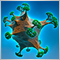5
7 394

‌‌

### Introduction

The first article Graphical Interfaces I: Preparation of the Library Structure (Chapter 1) considers in detail what this library is for. You will find a list of articles with links at the end of each chapter. There, you can also download a complete version of the library at the current stage of development. The files must be located in the same directories as in the archive.

We continue to develop the rendered table. Let us enumerate the new features to be added to it.

• Sorting the table data.
• Managing the number of columns and rows: adding and removing the columns and rows at a specified index; fully clearing the table (leaves only one column and one row); rebuilding the table (clears the table and sets new dimensions).
• Extension of the user management over the graphical interface: handling of a double click event is added.
• We will start adding controls to the table cells: checkboxes and buttons.

If you already create graphical interfaces with the help of this library and use tables of the CTable type to display data, you are now advised to move on to tables of the CCanvasTable type. Starting from this article, it is completely on par with tables of other types in this library, and surpasses them in some respects.

### Table sorting

Most methods for sorting the table data are the same as in the CTable. The article Graphical Interfaces X: Time control, List of checkboxes control and table sorting describes how all of that is arranged in detail. Here, the changes and additions related to tables of the CCanvasTable type will be mentioned briefly.

Drawing a sorted table sign will require a static array of the CTImage type with two elements. It will contain the paths to the images for displaying the sorting direction. If the user had not set a custom image, then the default icons will be used. Initialization with the default values and filling the image arrays are performed in the CCanvasTable::CreateHeaders() method for creating the headers.

```//+------------------------------------------------------------------+
//| Class for creating a rendered table                              |
//+------------------------------------------------------------------+
class CCanvasTable : public CElement
{
private:
//--- Icons for the sign of sorted data
CTImage           m_sort_arrows;
//---
public:
//--- Setting the icons for the sign of sorted data
void              SortArrowFileAscend(const string path)  { m_sort_arrows.m_bmp_path=path; }
void              SortArrowFileDescend(const string path) { m_sort_arrows.m_bmp_path=path; }
};
//+------------------------------------------------------------------+
//| Constructor                                                      |
//+------------------------------------------------------------------+
CCanvasTable::CCanvasTable(void)
{
...
//--- Initializing the structure of the sorting sign
m_sort_arrows.m_bmp_path="";
m_sort_arrows.m_bmp_path="";
}
//+------------------------------------------------------------------+
//| Creates the table headers                                        |
//+------------------------------------------------------------------+
{
//--- Leave, if the headers are disabled
return(true);
//--- Forming the object name
//--- Coordinates
int x =m_x+1;
int y =m_y+1;
//--- Define the icons as a sign of the possibility of sorting the table
if(m_sort_arrows.m_bmp_path=="")
m_sort_arrows.m_bmp_path="::Images\\EasyAndFastGUI\\Controls\\SpinInc.bmp";
if(m_sort_arrows.m_bmp_path=="")
m_sort_arrows.m_bmp_path="::Images\\EasyAndFastGUI\\Controls\\SpinDec.bmp";
//---
for(int i=0; i<2; i++)
{
::ResetLastError();
m_sort_arrows[i].m_image_width,m_sort_arrows[i].m_image_height))
{
::Print(__FUNCTION__," > error: ",::GetLastError());
}
}
//--- Creating the object
::ResetLastError();
{
::Print(__FUNCTION__," > Failed to create a canvas for drawing the table headers: ",::GetLastError());
return(false);
}
//--- Attach to the chart
//--- Set properties
//--- Coordinates
//--- Store the size
//--- Margins from the edge of the panel
//--- Store the object pointer
//--- Set the size of the visible area
//--- Set the frame offset within the image along the X and Y axes
...
return(true);
}
```

The CCanvasTable::DrawSignSortedData() method will be used for drawing the sign of a sorted array. This element is drawn only if (1) sorting mode is enabled and (2) table data sorting has already been performed. Offsets can be controlled using the CCanvasTable::SortArrowXGap() and CCanvasTable::SortArrowYGap() methods. The icon indicates that sorting in ascending order will have the index 0, and in descending order – 1. Then comes a double loop for drawing the image. This topic has already been discussed in detail.‌

```class CCanvasTable : public CElement
{
private:
//--- Offsets for the sign icon of sorted data
int               m_sort_arrow_x_gap;
int               m_sort_arrow_y_gap;
//---
public:
//--- Offsets for the sign of sorted table
void              SortArrowXGap(const int x_gap)          { m_sort_arrow_x_gap=x_gap;         }
void              SortArrowYGap(const int y_gap)          { m_sort_arrow_y_gap=y_gap;         }
//---
private:
//--- Draws the sign of the possibility of sorting the table
void              DrawSignSortedData(void);
};
//+------------------------------------------------------------------+
//| Constructor                                                      |
//+------------------------------------------------------------------+
CCanvasTable::CCanvasTable(void) : m_sort_arrow_x_gap(20),
m_sort_arrow_y_gap(6)
{
...
}
//+------------------------------------------------------------------+
//| Draws the sign of the possibility of sorting the table           |
//+------------------------------------------------------------------+
void CCanvasTable::DrawSignSortedData(void)
{
//--- Leave, if (1) sorting is disabled or (2) has not been performed yet
if(!m_is_sort_mode || m_is_sorted_column_index==WRONG_VALUE)
return;
//--- Calculating coordinates
int x =m_columns[m_is_sorted_column_index].m_x2-m_sort_arrow_x_gap;
int y =m_sort_arrow_y_gap;
//--- The selected icon for the sorting direction
int image_index=(m_last_sort_direction==SORT_ASCEND)? 0 : 1;
//--- Draw
for(uint ly=0,i=0; ly<m_sort_arrows[image_index].m_image_height; ly++)
{
for(uint lx=0; lx<m_sort_arrows[image_index].m_image_width; lx++,i++)
{
//--- If there is no color, go to the next pixel
if(m_sort_arrows[image_index].m_image_data[i]<1)
continue;
//--- Get the color of the lower layer (header background) and color of the specified pixel of the icon
uint pixel_color =m_sort_arrows[image_index].m_image_data[i];
//--- Blend the colors
uint foreground=::ColorToARGB(m_clr.BlendColors(background,pixel_color));
//--- Draw the pixel of the overlay icon
}
}
}
```

The CCanvasTable::Swap() method is used for swapping the table values while sorting. The difference here is that it is necessary to move not only the text and/or images displayed in the cell, but also a large number of cell properties. For convenience, and to eliminate duplicate code fragments, an auxiliary CCanvasTable::ImageCopy() method will be required when moving the images. It is passed two arrays of the CTImage type — receiver and source of the data. The index of the copied image is passed as the third argument, since a single cell may contain multiple images.

```class CCanvasTable : public CElement
{
private:
//--- Copies the image data from one array to another
void              ImageCopy(CTImage &destination[],CTImage &source[],const int index);
};
//+------------------------------------------------------------------+
//| Copies the image data from one array to another                  |
//+------------------------------------------------------------------+
void CCanvasTable::ImageCopy(CTImage &destination[],CTImage &source[],const int index)
{
//--- Copy the image pixels
::ArrayCopy(destination[index].m_image_data,source[index].m_image_data);
//--- Copy the image properties
destination[index].m_image_width  =source[index].m_image_width;
destination[index].m_image_height =source[index].m_image_height;
destination[index].m_bmp_path     =source[index].m_bmp_path;
}
```

The resulting code of the CCanvasTable::Swap() looks as shown in the listing below. Before moving the images, it is first necessary to check if they are there, and if not, then go to the next column. If one of the cells contains images, then they are moved using the CCanvasTable::ImageCopy() method. This operation is the same as in the case of moving other properties of the cell. That is, first store the property of the first cell. Then, this property is copied from the second cell to the place of the first cell. Finally, the previously stored value of the first cell is moved to the place of the second cell.

```class CCanvasTable : public CElement
{
private:
//--- Swap the values in the specified cells
void              Swap(uint r1,uint r2);
};
//+------------------------------------------------------------------+
//| Swap the elements                                                |
//+------------------------------------------------------------------+
void CCanvasTable::Swap(uint r1,uint r2)
{
//--- Iterate over all columns in a loop
for(uint c=0; c<m_columns_total; c++)
{
//--- Swap the full text
string temp_text                    =m_columns[c].m_rows[r1].m_full_text;
m_columns[c].m_rows[r1].m_full_text =m_columns[c].m_rows[r2].m_full_text;
m_columns[c].m_rows[r2].m_full_text =temp_text;
//--- Swap the short text
temp_text                            =m_columns[c].m_rows[r1].m_short_text;
m_columns[c].m_rows[r1].m_short_text =m_columns[c].m_rows[r2].m_short_text;
m_columns[c].m_rows[r2].m_short_text =temp_text;
//--- Swap the number of decimal places
uint temp_digits                 =m_columns[c].m_rows[r1].m_digits;
m_columns[c].m_rows[r1].m_digits =m_columns[c].m_rows[r2].m_digits;
m_columns[c].m_rows[r2].m_digits =temp_digits;
//--- Swap the text color
color temp_text_color                =m_columns[c].m_rows[r1].m_text_color;
m_columns[c].m_rows[r1].m_text_color =m_columns[c].m_rows[r2].m_text_color;
m_columns[c].m_rows[r2].m_text_color =temp_text_color;
//--- Swap the index of the selected icon
int temp_selected_image                  =m_columns[c].m_rows[r1].m_selected_image;
m_columns[c].m_rows[r1].m_selected_image =m_columns[c].m_rows[r2].m_selected_image;
m_columns[c].m_rows[r2].m_selected_image =temp_selected_image;
//--- Check if the cells contain images
int r1_images_total=::ArraySize(m_columns[c].m_rows[r1].m_images);
int r2_images_total=::ArraySize(m_columns[c].m_rows[r2].m_images);
//--- Go to the next column, if both cells have no images
if(r1_images_total<1 && r2_images_total<1)
continue;
//--- Swap the images
CTImage r1_temp_images[];
//---
::ArrayResize(r1_temp_images,r1_images_total);
for(int i=0; i<r1_images_total; i++)
ImageCopy(r1_temp_images,m_columns[c].m_rows[r1].m_images,i);
//---
::ArrayResize(m_columns[c].m_rows[r1].m_images,r2_images_total);
for(int i=0; i<r2_images_total; i++)
ImageCopy(m_columns[c].m_rows[r1].m_images,m_columns[c].m_rows[r2].m_images,i);
//---
::ArrayResize(m_columns[c].m_rows[r2].m_images,r1_images_total);
for(int i=0; i<r1_images_total; i++)
ImageCopy(m_columns[c].m_rows[r2].m_images,r1_temp_images,i);
}
}
```

Clicking one of the table headers calls the CCanvasTable::OnClickHeaders() method, which starts the data sorting. It is significantly simpler in this class than in a table of the CTable type: compare and see for yourselves.

```class CCanvasTable : public CElement
{
private:
//--- Handling clicking on a header
};
//+------------------------------------------------------------------+
//| Handling clicking on a header                                    |
//+------------------------------------------------------------------+
{
//--- Leave, if (1) the sorting mode is disabled or (2) in the process of changing the column width
if(!m_is_sort_mode || m_column_resize_control!=WRONG_VALUE)
return(false);
//--- Leave, if the scrollbar is active
if(m_scrollv.ScrollState() || m_scrollh.ScrollState())
return(false);
//--- Leave, if it has a different object name
return(false);
//--- For determining the column index
uint column_index=0;
//--- Get the relative X coordinate below the mouse cursor
for(uint c=0; c<m_columns_total; c++)
{
//--- If the header is found, store its index
if(x>=m_columns[c].m_x && x<=m_columns[c].m_x2)
{
column_index=c;
break;
}
}
//--- Sort the data according to the specified column
SortData(column_index);
//--- Send a message about it
::EventChartCustom(m_chart_id,ON_SORT_DATA,CElementBase::Id(),m_is_sorted_column_index,::EnumToString(DataType(column_index)));
return(true);
}
```

The remaining methods for sorting data are left unchanged and have no difference from the previously considered ones. Therefore, only a list with declarations of these fields and methods in the CCanvasTable class will be considered:‌

```class CCanvasTable : public CElement
{
private:
//--- Mode of sorting data according to columns
bool              m_is_sort_mode;
//--- Index of the sorted column (WRONG_VALUE – table is not sorted)
int               m_is_sorted_column_index;
//--- Last sorting direction
ENUM_SORT_MODE    m_last_sort_direction;
//---
public:
//--- Data sorting mode
void              IsSortMode(const bool flag)             { m_is_sort_mode=flag;              }

//--- Get/set the data type
ENUM_DATATYPE     DataType(const uint column_index);
void              DataType(const uint column_index,const ENUM_DATATYPE type);
//--- Sort the data according to the specified column
void              SortData(const uint column_index=0);
//---
private:
//--- Handling clicking on a header

//--- Quicksort method
void              QuickSort(uint beg,uint end,uint column,const ENUM_SORT_MODE mode=SORT_ASCEND);
//--- Checking the sorting conditions
bool              CheckSortCondition(uint column_index,uint row_index,const string check_value,const bool direction);
};

```

Sorting in this type of tables is demonstrated below: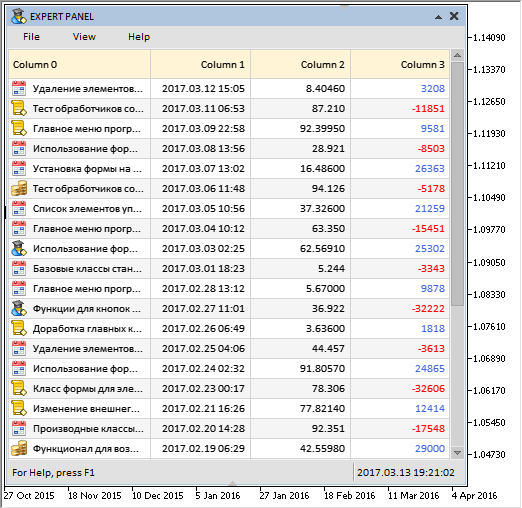Fig. 1. Demonstration of sorting in tables of the CCanvasTable type.‌

### Adding and removing columns and rows

One of the previous articles has considered methods for adding and removing columns and rows for tables of the CTable type. That version allowed adding only at the end of the table. This nuisance will now be fixed: let us make adding a column or row possible with indication of the index to be added at.

Example: add a column to the beginning of a table with data: that is, the new column must become the first (index 0). The array of columns must be increased by one element, while the properties and values of all table cells must be shifted one cell to the right, leaving only the cells of the new column blank. The same principle applies when adding rows to the table.

The reverse principle works when it is necessary to remove a column or row. Let us try to remove the first column from the previous example: first, the data and properties of the cells will be shifted left by one element, and after that, the size of the columns array will be decreased by one element.

Here, auxiliary methods have been implemented in order to facilitate creating the methods for adding and removing the columns and rows. The CCanvasTable::ColumnInitialize() and CCanvasTable::CellInitialize() methods will be used to initialize the cells, added columns and rows with the default values.

```class CCanvasTable : public CElement
{
private:
//--- Initialize the specified column with the default values
void              ColumnInitialize(const uint column_index);
//--- Initialize the specified cell with the default values
void              CellInitialize(const uint column_index,const uint row_index);
};
//+------------------------------------------------------------------+
//| Initialize the specified column with the default values          |
//+------------------------------------------------------------------+
void CCanvasTable::ColumnInitialize(const uint column_index)
{
//--- Initialize the column properties with the default values
m_columns[column_index].m_x              =0;
m_columns[column_index].m_x2             =0;
m_columns[column_index].m_width          =100;
m_columns[column_index].m_type           =TYPE_STRING;
m_columns[column_index].m_text_align     =ALIGN_CENTER;
m_columns[column_index].m_text_x_offset  =m_text_x_offset;
m_columns[column_index].m_image_x_offset =m_image_x_offset;
m_columns[column_index].m_image_y_offset =m_image_y_offset;
}
//+------------------------------------------------------------------+
//| Initialize the specified cell with the default values            |
//+------------------------------------------------------------------+
void CCanvasTable::CellInitialize(const uint column_index,const uint row_index)
{
m_columns[column_index].m_rows[row_index].m_full_text      ="";
m_columns[column_index].m_rows[row_index].m_short_text     ="";
m_columns[column_index].m_rows[row_index].m_selected_image =0;
m_columns[column_index].m_rows[row_index].m_text_color     =m_cell_text_color;
m_columns[column_index].m_rows[row_index].m_digits         =0;
m_columns[column_index].m_rows[row_index].m_type           =CELL_SIMPLE;
//--- By default, the cells do not contain images
::ArrayFree(m_columns[column_index].m_rows[row_index].m_images);
}
```

To copy the properties of a column to another column, the CCanvasTable::ColumnCopy() method must be used. Its code is provided in the listing below:

```class CCanvasTable : public CElement
{
private:
//--- Makes a copy of the specified column (source) to a new location (dest.)
void              ColumnCopy(const uint destination,const uint source);
};
//+------------------------------------------------------------------+
//| Makes a copy of specified column (source) to new location (dest.)|
//+------------------------------------------------------------------+
void CCanvasTable::ColumnCopy(const uint destination,const uint source)
{
m_columns[destination].m_width          =m_columns[source].m_width;
m_columns[destination].m_type           =m_columns[source].m_type;
m_columns[destination].m_text_align     =m_columns[source].m_text_align;
m_columns[destination].m_text_x_offset  =m_columns[source].m_text_x_offset;
m_columns[destination].m_image_x_offset =m_columns[source].m_image_x_offset;
m_columns[destination].m_image_y_offset =m_columns[source].m_image_y_offset;
}
```

The CCanvasTable::CellCopy() method copies a specified cell to another. To do this, it is necessary to pass the column and row indexes of the receiver cell and column and row indexes of the source cell.‌

```class CCanvasTable : public CElement
{
private:
//--- Makes a copy of the specified cell (source) to a new location (dest.)
void              CellCopy(const uint column_dest,const uint row_dest,const uint column_source,const uint row_source);
};
//+------------------------------------------------------------------+
//| Makes a copy of specified cell (source) to new location (dest.)  |
//+------------------------------------------------------------------+
void CCanvasTable::CellCopy(const uint column_dest,const uint row_dest,const uint column_source,const uint row_source)
{
m_columns[column_dest].m_rows[row_dest].m_type           =m_columns[column_source].m_rows[row_source].m_type;
m_columns[column_dest].m_rows[row_dest].m_digits         =m_columns[column_source].m_rows[row_source].m_digits;
m_columns[column_dest].m_rows[row_dest].m_full_text      =m_columns[column_source].m_rows[row_source].m_full_text;
m_columns[column_dest].m_rows[row_dest].m_short_text     =m_columns[column_source].m_rows[row_source].m_short_text;
m_columns[column_dest].m_rows[row_dest].m_text_color     =m_columns[column_source].m_rows[row_source].m_text_color;
m_columns[column_dest].m_rows[row_dest].m_selected_image =m_columns[column_source].m_rows[row_source].m_selected_image;
//--- Copy the array size from the source to receiver
int images_total=::ArraySize(m_columns[column_source].m_rows[row_source].m_images);
::ArrayResize(m_columns[column_dest].m_rows[row_dest].m_images,images_total);
//---
for(int i=0; i<images_total; i++)
{
//--- Copy, if there are images
if(::ArraySize(m_columns[column_source].m_rows[row_source].m_images[i].m_image_data)<1)
continue;
//--- make a copy of the image
ImageCopy(m_columns[column_dest].m_rows[row_dest].m_images,m_columns[column_source].m_rows[row_source].m_images,i);
}
}
```

As part of eliminating the repeated code from the method, another simple method has been implemented inside this method, which will be called after the changes made in rebuilding of the table. Each time rows and columns are added and removed, the table must be recalculated and resized, and after that, the table must be redrawn to reflect these changes. The CCanvasTable::RecalculateAndResizeTable() method will be used for this purpose. Here, the sole parameter indicates if it is necessary to fully redraw the table (true) or simply update it (false).

```class CCanvasTable : public CElement
{
private:
//--- Recalculate with consideration of the recent changes and resize the table
void              RecalculateAndResizeTable(const bool redraw=false);
};
//+------------------------------------------------------------------+
//| Calculate with consideration of recent changes and resize table  |
//+------------------------------------------------------------------+
void CCanvasTable::RecalculateAndResizeTable(const bool redraw=false)
{
//--- Calculate the table sizes
CalculateTableSize();
//--- Resize the table
ChangeTableSize();
//--- Update the table
UpdateTable(redraw);
}
```

The methods for adding and removing rows and columns are much simpler and easier to understand than the versions of these methods in tables of the CTable type. You can compare the code of these methods on your own. Here, only the code of the methods for adding and removing columns will be given as an example. The code for working with the rows has the same sequence of actions as described previously in the beginning of this section. Please note: the sign of sorted table will also move together with the sorted column both when adding and when removing. In the case when a sorted column is removed, the field containing the index of the sorted column is zeroed.‌

```class CCanvasTable : public CElement
{
public:
//--- Adds a column to the table at the specified index
void              AddColumn(const int column_index,const bool redraw=false);
//--- Removes a column from the table at the specified index
void              DeleteColumn(const int column_index,const bool redraw=false);
};
//+------------------------------------------------------------------+
//| Adds a column to the table at the specified index                |
//+------------------------------------------------------------------+
void CCanvasTable::AddColumn(const int column_index,const bool redraw=false)
{
//--- Increase the array size by one element
int array_size=(int)ColumnsTotal();
m_columns_total=array_size+1;
::ArrayResize(m_columns,m_columns_total);
//--- Set the size of the rows arrays
::ArrayResize(m_columns[array_size].m_rows,m_rows_total);
//--- Adjustment of the index in case the range has been exceeded
int checked_column_index=(column_index>=(int)m_columns_total)? (int)m_columns_total-1 : column_index;
//--- Shift other columns (starting from the end of the array to the index of the added column)
for(int c=array_size; c>=checked_column_index; c--)
{
//--- Shift the sign of sorted array
if(c==m_is_sorted_column_index && m_is_sorted_column_index!=WRONG_VALUE)
m_is_sorted_column_index++;
//--- Index of the previous column
int prev_c=c-1;
//--- Initialize the new column with the default values
if(c==checked_column_index)
ColumnInitialize(c);
//--- Move the data from the previous column to the current column
else
ColumnCopy(c,prev_c);
//---
for(uint r=0; r<m_rows_total; r++)
{
//--- Initialize the new column cells with the default values
if(c==checked_column_index)
{
CellInitialize(c,r);
continue;
}
//--- Move the data from the previous column cell to the current column cell
CellCopy(c,r,prev_c,r);
}
}
//--- Calculate and resize to the table
RecalculateAndResizeTable(redraw);
}
//+------------------------------------------------------------------+
//| Removes a column from the table at the specified index           |
//+------------------------------------------------------------------+
void CCanvasTable::DeleteColumn(const int column_index,const bool redraw=false)
{
//--- Get the size of the array of columns
int array_size=(int)ColumnsTotal();
//--- Adjustment of the index in case the range has been exceeded
int checked_column_index=(column_index>=array_size)? array_size-1 : column_index;
//--- Shift other columns (starting from the specified index to the last column)
for(int c=checked_column_index; c<array_size-1; c++)
{
//--- Shift the sign of sorted array
if(c!=checked_column_index)
{
if(c==m_is_sorted_column_index && m_is_sorted_column_index!=WRONG_VALUE)
m_is_sorted_column_index--;
}
//--- Zero, if a sorted column was removed
else
m_is_sorted_column_index=WRONG_VALUE;
//--- Index of the next column
int next_c=c+1;
//--- Move the data from the next column to the current column
ColumnCopy(c,next_c);
//--- Move the data from the next column cells to the current column cells
for(uint r=0; r<m_rows_total; r++)
CellCopy(c,r,next_c,r);
}
//--- Decrease the array of columns by one element
m_columns_total=array_size-1;
::ArrayResize(m_columns,m_columns_total);
//--- Calculate and resize to the table
RecalculateAndResizeTable(redraw);
}
```

The methods for fully rebuilding and clearing the table are also very simple, unlike the similar methods in tables of the CTable type. Here, it is sufficient to set the new size by calling the CCanvasTable::TableSize() method. The minimum size is set when clearing the table, leaving only one column and one row. The fields containing the values of the selected row, sorting direction and index of the sorted column are also zeroed when clearing. The new table sizes are calculated and set at the end of both methods.

```class CCanvasTable : public CElement
{
public:
//--- Rebuilding the table
void              Rebuilding(const int columns_total,const int rows_total,const bool redraw=false);
//--- Clears the table. Only one column and one row are left.
void              Clear(const bool redraw=false);
};
//+------------------------------------------------------------------+
//| Rebuilding the table                                             |
//+------------------------------------------------------------------+
void CCanvasTable::Rebuilding(const int columns_total,const int rows_total,const bool redraw=false)
{
//--- Set the new size
TableSize(columns_total,rows_total);
//--- Calculate and resize to the table
RecalculateAndResizeTable(redraw);
}
//+------------------------------------------------------------------+
//| Clears the table. Only one column and one row are left.          |
//+------------------------------------------------------------------+
void CCanvasTable::Clear(const bool redraw=false)
{
//--- Set the minimum size of 1x1
TableSize(1,1);
//--- Set the default values
m_selected_item_text     ="";
m_selected_item          =WRONG_VALUE;
m_last_sort_direction    =SORT_ASCEND;
m_is_sorted_column_index =WRONG_VALUE;
//--- Calculate and resize to the table
RecalculateAndResizeTable(redraw);
}
```

This is how it works: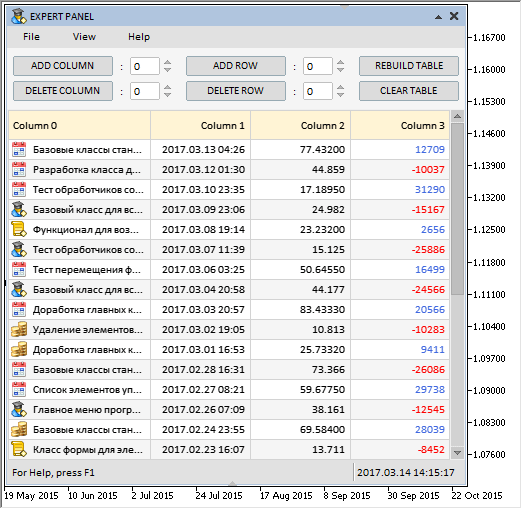Fig. 2. Demonstration of managing the table dimensions.

The test application featured in the animation above can be downloaded at the end of the article.

### Left mouse button double click event

Sometimes it may be necessary to call a certain event by a double click. Let us implement the generation of such an event in the library. To do this, add the ON_DOUBLE_CLICK identifier for the left mouse button double click event to the Defines.mqh file:

```//+------------------------------------------------------------------+
//|                                                      Defines.mqh |
//|                        Copyright 2015, MetaQuotes Software Corp. |
//|                                              http://www.mql5.com |
//+------------------------------------------------------------------+
...
#define ON_DOUBLE_CLICK             (34) // Left mouse button double click
...
```

Event with the ON_DOUBLE_CLICK identifier will be generated in the CMouse class. In operating systems, this event is usually generated by the “press-release-press” actions of the left mouse button. But testing in the terminal environment has shown that is not always possible to immediately trace an event of pressing the left mouse button with the arrival of the CHARTEVENT_MOUSE_MOVE event (parameter sparam). It was therefore decided to implement the generation of the event through the “press-release-press-release” actions. These actions can be accurately tracked with the arrival of the CHARTEVENT_CLICK event.

By default, at least 300 milliseconds must pass between two clicks. For tracking the CHARTEVENT_CLICK event, the CMouse::CheckDoubleClick() method will be called in the mouse event handler. Two static variables are declared at the beginning of this method, where the values of the previous and current tick (the number of milliseconds that have passed since the start of the system) will be stored at each call to the method. If the number of milliseconds between these values is less than specified in the m_pause_between_clicks field, then the left mouse button double click event will be generated. ‌

```//+------------------------------------------------------------------+
//| Class for getting the mouse parameters                           |
//+------------------------------------------------------------------+
class CMouse
{
private:
//--- Pause button the left mouse button clicks (for determining the double click)
uint              m_pause_between_clicks;
//---
private:
//--- Checking the left mouse button double click
bool              CheckDoubleClick(void);
};
//+------------------------------------------------------------------+
//| Constructor                                                      |
//+------------------------------------------------------------------+
CMouse::CMouse(void) : m_pause_between_clicks(300)
{
...
}
//+------------------------------------------------------------------+
//| Handling mouse events                                            |
//+------------------------------------------------------------------+
void CMouse::OnEvent(const int id,const long &lparam,const double &dparam,const string &sparam)
{
...
//--- Handling event of clicking on the chart
if(id==CHARTEVENT_CLICK)
{
//--- Check the left mouse button double click
CheckDoubleClick();
return;
}
}
//+------------------------------------------------------------------+
//| Checking the left mouse button double click                      |
//+------------------------------------------------------------------+
void CMouse::CheckDoubleClick(void)
{
static uint prev_depressed =0;
static uint curr_depressed =::GetTickCount();
//--- Update the values
prev_depressed =curr_depressed;
curr_depressed =::GetTickCount();
//--- Determine the time between the clicks
uint counter=curr_depressed-prev_depressed;
//--- If the time passed between the two clicks is less than specified, send a message about a double click
if(counter<m_pause_between_clicks)
::EventChartCustom(m_chart.ChartId(),ON_DOUBLE_CLICK,counter,0.0,"");
}
```

Thus, the event handlers of all library classes allow tracking the left mouse button double clicks anywhere in the chart area, regardless of whether there is a graphical object under the cursor.

### Controls in the table cells

This article will start the topic of controls in table cells. For example, this feature may be needed when it is necessary to create a multi-parameter expert system. It is convenient to implement the graphical interface of this system as a table. Let us start adding such controls to the table cells with the checkboxes and buttons.

First of all, this will require a new ENUM_TYPE_CELL enumeration, where the cell types will be defined:

```//+------------------------------------------------------------------+
//| Enumeration of the table cell types                              |
//+------------------------------------------------------------------+
enum ENUM_TYPE_CELL
{
CELL_SIMPLE   =0,
CELL_BUTTON   =1,
CELL_CHECKBOX =2
};
```

By default, during the initialization the table cells are assigned the simple type – CELL_SIMPLE, which means “cell without control”. To set or get the cell type, use the CCanvasTable::CellType() methods, their code is provided in the listing below. If cells are assigned the CELL_CHECKBOX (checkbox) type, it is also necessary to set images for the checkbox states. The minimum number of images for this cell type is two. It is possible to set more than two icons, this will allow working with multiparameter checkboxes.

```class CCanvasTable : public CElement
{
//--- Setting/getting the cell type
void              CellType(const uint column_index,const uint row_index,const ENUM_TYPE_CELL type);
ENUM_TYPE_CELL    CellType(const uint column_index,const uint row_index);
};
//+------------------------------------------------------------------+
//| Sets the cell type                                               |
//+------------------------------------------------------------------+
void CCanvasTable::CellType(const uint column_index,const uint row_index,const ENUM_TYPE_CELL type)
{
//--- Checking for exceeding the array range
if(!CheckOutOfRange(column_index,row_index))
return;
//--- Set the cell type
m_columns[column_index].m_rows[row_index].m_type=type;
}
//+------------------------------------------------------------------+
//| Gets the cell type                                               |
//+------------------------------------------------------------------+
ENUM_TYPE_CELL CCanvasTable::CellType(const uint column_index,const uint row_index)
{
//--- Checking for exceeding the array range
if(!CheckOutOfRange(column_index,row_index))
return(WRONG_VALUE);
//--- Return the data type for the specified column
return(m_columns[column_index].m_rows[row_index].m_type);
}
```

Series of images for checkboxes and buttons can have different sizes, therefore, it is necessary to have the ability to set the X and Y offsets of the images for each column separately. This can bee done using the CCanvasTable::ImageXOffset() and CCanvasTable::ImageYOffset() methods:

```class CCanvasTable : public CElement
{
public:
//--- Offset of the images along the X and Y axes
void              ImageXOffset(const int &array[]);
void              ImageYOffset(const int &array[]);
};
```

Another addition is the mode for disabling deselection of a row when clicked again. This mode works only if the row selection mode is enabled.

```class CCanvasTable : public CElement
{
private:
//--- No deselection of row when clicked again
bool              m_is_without_deselect;
//---
public:
//--- The "No deselection of row when clicked again" mode
void              IsWithoutDeselect(const bool flag)   { m_is_without_deselect=flag;      }
};
```

Separate CCanvasTable::PressedRowIndex() and CCanvasTable::PressedCellColumnIndex() methods are now used for determining the index of the clicked column and row. They have been considered before as blocks of the CCanvasTable::OnClickTable() method. Their full code with new additions can be found in the files attached to the article. It must be separately noted that using these two methods together helps to determine the cell clicked by the left mouse button. Next, consider where the received column and row indexes are to be passed.

```class CCanvasTable : public CElement
{
private:
//--- Returns the index of the clicked row
int               PressedRowIndex(void);
//--- Returns the index of the clicked cell column
int               PressedCellColumnIndex(void);
};
```

When a table cell is clicked, it is necessary to get its type, and if it contains a control, then it is also necessary to determine if it is activated. For this purpose, a number of private methods has been implemented, the main of which is CCanvasTable::CheckCellElement(). It is passed the column and row indexes received from the CCanvasTable::PressedRowIndex() and CCanvasTable::PressedCellColumnIndex() methods. The method has two modes. Depending on the event type handled when the method is called, the third parameters is used, specifying if the event is a double click.

After that, the cell type is checked, and the appropriate method is called depending on its type. If a cell contains a “Button” control, then the CCanvasTable::CheckPressedButton() method will be called. The CCanvasTable::CheckPressedCheckBox() method is intended for cells with checkboxes.‌

```class CCanvasTable : public CElement
{
private:
//--- Check if the cell control was activated when clicked
bool              CheckCellElement(const int column_index,const int row_index,const bool double_click=false);
};
//+------------------------------------------------------------------+
//| Check if the cell control was activated when clicked             |
//+------------------------------------------------------------------+
bool CCanvasTable::CheckCellElement(const int column_index,const int row_index,const bool double_click=false)
{
//--- Leave, if the cell has no controls
if(m_columns[column_index].m_rows[row_index].m_type==CELL_SIMPLE)
return(false);
//---
switch(m_columns[column_index].m_rows[row_index].m_type)
{
//--- If it is a button cell
case CELL_BUTTON :
{
if(!CheckPressedButton(column_index,row_index,double_click))
return(false);
//---
break;
}
//--- If it is a checkbox cell
case CELL_CHECKBOX :
{
if(!CheckPressedCheckBox(column_index,row_index,double_click))
return(false);
//---
break;
}
}
//---
return(true);
}
```

Let us see the structure of the CCanvasTable::CheckPressedButton() and CCanvasTable::CheckPressedCheckBox() methods. The number of images in the cell is checked at the very beginning of both methods. Button cells must contain at least one icon, and checkbox cells at least two. Then it is necessary to determine if it is the image that was clicked. In the case of the checkbox, implement two ways for toggling it. A single click will only work if the icon with the checkbox is clicked. A double click anywhere in the cell toggles the checkbox. Both methods generate an event with the identifier corresponding to the control, provided that all conditions are met. The index of the image is passed as the double parameter, and the string parameter forms a string with the column and row indexes.

```class CCanvasTable : public CElement
{
private:
//--- Check if the button in the cell was clicked
bool              CheckPressedButton(const int column_index,const int row_index,const bool double_click=false);
//--- Check if the checkbox in the cell was clicked
bool              CheckPressedCheckBox(const int column_index,const int row_index,const bool double_click=false);
};
//+------------------------------------------------------------------+
//| Check if the button in the cell was clicked                      |
//+------------------------------------------------------------------+
bool CCanvasTable::CheckPressedButton(const int column_index,const int row_index,const bool double_click=false)
{
//--- Leave, if there are no images in the cell
if(ImagesTotal(column_index,row_index)<1)
{
::Print(__FUNCTION__," > Assign at least one image to the button cell!");
return(false);
}
//--- Get the relative coordinates under the mouse cursor
int x=m_mouse.RelativeX(m_table);
// --- Get the right border of the image
int image_x  =int(m_columns[column_index].m_x+m_columns[column_index].m_image_x_offset);
int image_x2 =int(image_x+m_columns[column_index].m_rows[row_index].m_images.m_image_width);
//--- Leave, if the click was not on the image
if(x>image_x2)
return(false);
else
{
//--- If this is not a double click, send a message
if(!double_click)
{
int image_index=m_columns[column_index].m_rows[row_index].m_selected_image;
::EventChartCustom(m_chart_id,ON_CLICK_BUTTON,CElementBase::Id(),image_index,string(column_index)+"_"+string(row_index));
}
}
//---
return(true);
}
//+------------------------------------------------------------------+
//| Check if the checkbox in the cell was clicked                    |
//+------------------------------------------------------------------+
bool CCanvasTable::CheckPressedCheckBox(const int column_index,const int row_index,const bool double_click=false)
{
//--- Leave, if there are no images in the cell
if(ImagesTotal(column_index,row_index)<2)
{
::Print(__FUNCTION__," > Assign at least two images to the checkbox cell!");
return(false);
}
//--- Get the relative coordinates under the mouse cursor
int x=m_mouse.RelativeX(m_table);
// --- Get the right border of the image
int image_x  =int(m_columns[column_index].m_x+m_image_x_offset);
int image_x2 =int(image_x+m_columns[column_index].m_rows[row_index].m_images.m_image_width);
//--- Leave, if (1) the click was not on the image and (2) it is not a double click
if(x>image_x2 && !double_click)
return(false);
else
{
//--- Current index of the selected image
int image_i=m_columns[column_index].m_rows[row_index].m_selected_image;
//--- Determine the next index for the image
int next_i=(image_i<ImagesTotal(column_index,row_index)-1)? ++image_i : 0;
//--- Select the next image and update the table
ChangeImage(column_index,row_index,next_i,true);
m_table.Update(false);
//--- Send a message about it
::EventChartCustom(m_chart_id,ON_CLICK_CHECKBOX,CElementBase::Id(),next_i,string(column_index)+"_"+string(row_index));
}
//---
return(true);
}
```

As a result, the code of the CCanvasTable::OnClickTable() and CCanvasTable::OnDoubleClickTable() methods for handling clicking on the table has become much more understandable and readable (see the code below). A corresponding event is generated depending on the modes enabled and the cell type that was clicked.

```class CCanvasTable : public CElement
{
private:
//--- Handling clicking on the table
bool              OnClickTable(const string clicked_object);
//--- Handling double clicking on the table
bool              OnDoubleClickTable(const string clicked_object);
};
//+------------------------------------------------------------------+
//| Handling clicking on the table                                   |
//+------------------------------------------------------------------+
bool CCanvasTable::OnClickTable(const string clicked_object)
{
//--- Leave, if (1) row selection mode is disabled or (2) in the process of changing the column width
if(m_column_resize_control!=WRONG_VALUE)
return(false);
//--- Leave, if the scrollbar is active
if(m_scrollv.ScrollState() || m_scrollh.ScrollState())
return(false);
//--- Leave, if it has a different object name
if(m_table.Name()!=clicked_object)
return(false);
//--- Determine the clicked row
int r=PressedRowIndex();
//--- Determine the clicked cell
int c=PressedCellColumnIndex();
//--- Check if the control in the cell was activated
bool is_cell_element=CheckCellElement(c,r);
//--- If (1) the row selection mode is enabled and (2) cell control is not activated
if(m_selectable_row && !is_cell_element)
{
//--- Change the color
RedrawRow(true);
m_table.Update();
//--- Send a message about it
::EventChartCustom(m_chart_id,ON_CLICK_LIST_ITEM,CElementBase::Id(),m_selected_item,string(c)+"_"+string(r));
}
//---
return(true);
}
//+------------------------------------------------------------------+
//| Handling double clicking on the table                            |
//+------------------------------------------------------------------+
bool CCanvasTable::OnDoubleClickTable(const string clicked_object)
{
if(!m_table.MouseFocus())
return(false);
//--- Determine the clicked row
int r=PressedRowIndex();
//--- Determine the clicked cell
int c=PressedCellColumnIndex();
//--- Check if the control in the cell was activated and return the result
return(CheckCellElement(c,r,true));
}
```

Events of left mouse button double click on a cell are handled in the control's event handler at the ON_DOUBLE_CLICK event:

```//+------------------------------------------------------------------+
//| Event handler                                                    |
//+------------------------------------------------------------------+
void CCanvasTable::OnEvent(const int id,const long &lparam,const double &dparam,const string &sparam)
{
...
//--- Handling the left mouse button double click
if(id==CHARTEVENT_CUSTOM+ON_DOUBLE_CLICK)
{
//--- Clicking the table
if(OnDoubleClickTable(sparam))
return;
//---
return;
}
}
```

In the end, everything works like this: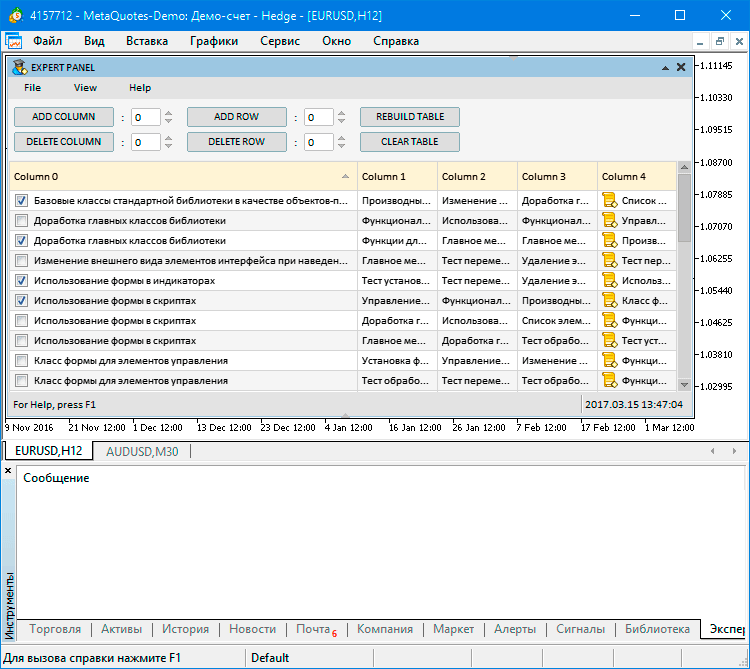Fig. 3. Demonstration of interaction with controls in the table cells.

### Conclusion

Currently, the general schematic of the library for creating graphical interfaces looks as shown below: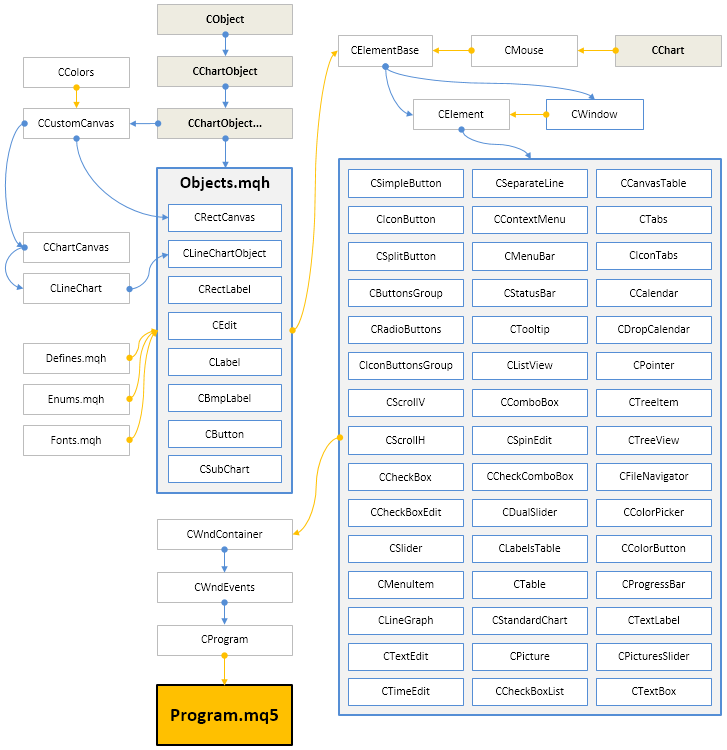Fig. 4. Structure of the library at the current stage of development.

If you have questions on using the material presented in those files, you can refer to the detailed description of the library development in one of the articles of this series or ask your question in the comments of this article.

Translated from Russian by MetaQuotes Software Corp.
Original article: https://www.mql5.com/ru/articles/3104

Attached files |
Last comments | Go to discussion (5)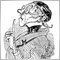| 27 Apr 2017 at 08:29

Hi,

your table project is really interesting and an example of good OOP coding.

May I ask whether you will be willing to think about an - I hope for other too - a very helpful add-on for complicated multi-symbol EAs?

Imagine an EA that trades many symbols with a lot of indicators with - for each symbol - an individual indicator setup of parameters (simple e.g.):

input string Sym1 = "EURUSD";

input int     Sym1MACDema1 = 12;

input int     Sym1MACDema2 = 26;

input int     Sym1MACDsma3 = 9

input ENUM_APPLIED_PRICE Sym1MACDprc =PRICE_CLOSE;

input string Sym2 = "GBPUSD";

input int     Sym2MACDema1 = 11;

input int     Sym2MACDema2 = 25;

input int     Sym2MACDsma3 = 10

input ENUM_APPLIED_PRICE  Sym2MACDprc =PRICE_CLOSE;

...

This would be a lot easier to handle for the user if this setup is presented as a table instead of a long list of parameters.

Especially if you enable your app to manage the StartegyTester by that csv-file. I think one has to manage the EA in the StrategyTester by the csv-file in the OnInit()-function.

For this one could add what the StratTester offers: defining a range. from, to, step.

But there the problem could be that in case of the Genetic Algorithm if OnInit() returns INIT_PARAMETERS_INCORRECT or INIT_FAILED the genetic algorithm counts this as a valid pass which will reduce the amount of really passed test runs and that reduces the validity if the final outcome.

I realized this problem with mt4 and I wrote this to the Service Desk - but I have no idea whether they have solved this or not.

Anyway good work - keep on :)| 27 Apr 2017 at 10:49
Carl Schreiber:

...

Thanks for the feedback and suggestion! I'll think about how this can be realized.| 3 May 2017 at 00:39

Hello, How can I programmatically move the main form or dialog to a specific location.

For example I would like to move the dialog to the left upper corner when the form title is clicked.| 28 May 2017 at 10:36

Hi!

This is a great library!

Do you still support MT4?

Having both MT4 and MT5 support would be a great way to be able to have a smooth transition from MT4 to MT5 when it is time for that.| 8 Jun 2017 at 15:21

Hello,

If i don't create menus, like in the code i modified below, i can't sort the table and single click on checkbox, why is this happening? @Anatoli Kazharski

```//+------------------------------------------------------------------+
//| Creates a trading panel                                          |
//+------------------------------------------------------------------+
bool CProgram::CreateExpertPanel(void)
{
//--- Creating form 1 for controls
if(!CreateWindow1("EXPERT PANEL"))
return(false);
/*/--- Creating controls:
return(false);
return(false);
return(false);
return(false);
return(false);*/
//--- Creating the status bar
if(!CreateStatusBar(1,25))
return(false);
//--- Buttons
return(false);
if(!CreateSimpleButton2(7,75,"DELETE COLUMN"))
return(false);
return(false);
if(!CreateSimpleButton4(180,75,"DELETE ROW"))
return(false);
if(!CreateSimpleButton5(353,50,"REBUILD TABLE"))
return(false);
if(!CreateSimpleButton6(353,75,"CLEAR TABLE"))
return(false);
//--- Edits
if(!CreateSpinEdit1(114,52,":"))
return(false);
if(!CreateSpinEdit2(114,77,":"))
return(false);
if(!CreateSpinEdit3(287,52,":"))
return(false);
if(!CreateSpinEdit4(287,77,":"))
return(false);
//--- Create rendered table
if(!CreateCanvasTable(1,103)) // 103 | 42
return(false);
//--- Redrawing of the chart
m_chart.Redraw();
return(true);
}
```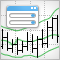A Universal Channel with the Graphical Interface

All channel indicators are displayed as three lines, including central, top and bottom lines. The drawing principle of the central line is similar to a moving average, while the moving average indicator is mostly used for drawing channels. The top and bottom lines are located at equal distances from the central line. This distance can be determined in points, as percent of price (the Envelopes indicator), using a standard deviation value (Bollinger Bands), or an ATR value (Keltner channel).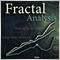Calculating the Hurst exponent

The article thoroughly explains the idea behind the Hurst exponent, as well as the meaning of its values and the calculation algorithm. A number of financial market segments are analyzed and the method of working with MetaTrader 5 products implementing the fractal analysis is described.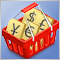Patterns available when trading currency baskets. Part II

We continue our discussion of the patterns traders can come across while trading currency baskets. In this part, we will consider the patterns formed when using combined trend indicators. Indicators based on a currency index are to be used as the analytical tool.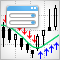Universal Trend with the Graphical Interface

In this article a universal trend indicator is created based on a number of standard indicators. An additionally created graphical interface allows selecting the type of indicator and adjusting its parameter. The indicator is displayed in a separate window with rows of colored icons.